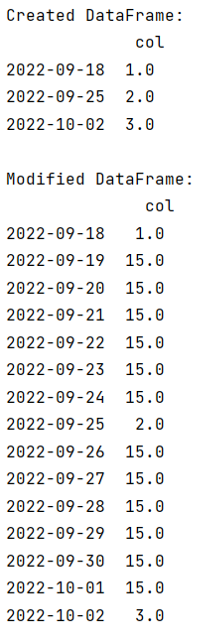# Pandas DataFrame asfreq() Method with Example

DataFrame.asfreq() Method: Learn about the Pandas DataFrame asfreq() Method with its usages, syntax, and examples.
Submitted by Pranit Sharma, on September 17, 2022

Pandas is a special tool that allows us to perform complex manipulations of data effectively and efficiently. Inside pandas, we mostly deal with a dataset in the form of DataFrame. DataFrames are 2-dimensional data structures in pandas. DataFrames consist of rows, columns, and data.

## pandas.DataFrame.asfreq() Method

Pandas DataFrame.asfreq() method is used to convert Time-Series to a specific frequency. This function enables us to frame/fill back the missing values. It Returns the original data obeying a new index with the specified frequency. This method is useful when we perform operations like summarization, which can be necessary to represent the data at the new frequency.

## pandas.DataFrame.asfreq() Syntax

```DataFrame.asfreq(
freq,
method=None,
how=None,
normalize=False,
fill_value=None
)
```

## pandas.DataFrame.asfreq() Parameters

• freq: String or object
• method: specific method to fill the holes.
• how: For PeriodIndex only (see PeriodIndex.asfreq).
• normalize: option to reset the index or not
• fill_value: values to be replaced with.

Let us understand with the help of an example,

## Pandas DataFrame asfreq() Method Example

```# Importing pandas package
import pandas as pd

# Creating dictionary
d = {'col':[1.0,2.0,3.0]}

# Creating DataFrame
df = pd.DataFrame(d,index=pd.date_range('16/09/2022',
periods=3,freq='W'))

# Display Original DataFrames
print("Created DataFrame:\n",df,"\n")

# Using asfreq method
df = df.asfreq(freq ='D', fill_value = 15.0)

# Display modified DataFrame
print("Modified DataFrame:\n",df)
```

Output:Reference:

What's New (MCQs)

Top Interview Coding Problems/Challenges!

Languages: » C » C++ » C++ STL » Java » Data Structure » C#.Net » Android » Kotlin » SQL
Web Technologies: » PHP » Python » JavaScript » CSS » Ajax » Node.js » Web programming/HTML
Solved programs: » C » C++ » DS » Java » C#
Aptitude que. & ans.: » C » C++ » Java » DBMS
Interview que. & ans.: » C » Embedded C » Java » SEO » HR
CS Subjects: » CS Basics » O.S. » Networks » DBMS » Embedded Systems » Cloud Computing
» Machine learning » CS Organizations » Linux » DOS
More: » Articles » Puzzles » News/Updates

© https://www.includehelp.com some rights reserved.